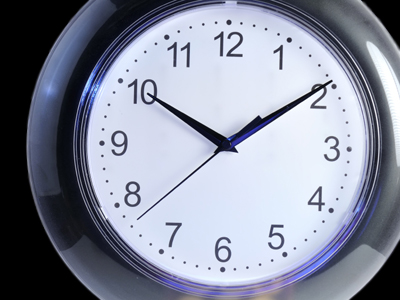The numbers on a clock represent 5 minutes so when the minute hand points to the number 2 then it is 10 minutes past the hour.

# Year 2 Time - Telling the Time to 5 Minutes

This quiz addresses the requirements of the National Curriculum KS1 Maths and Numeracy for children aged 6 and 7 in year 2. Specifically this quiz is aimed at the section dealing with telling the time to 5 minutes.

Once children in Year 2 are familiar with telling the time to the hour, half hour and quarter hour, they begin to recognise how to tell the time to 5 minutes. This may be linked to their work on the five times table, as they link this to counting around the clock in 5s to work out what time it is to five minutes.

To see a larger image, click on the picture.
Question 1
How many minutes to 7?
7
20
10
50
It is 10 minutes until the minute hand is back at the top
Question 2
What time is this?
Ten to ten
Two minutes past ten
Ten to two
Ten past ten
The minute hand is 10 minutes past the hour
Question 3
What time will it be in 5 minutes?
One minute past ten
Five past five
Ten past five
Five past ten
In 5 minutes, the minute hand will have moved to the 1, which shows us five past the hour
Question 4
How many minutes to 12?
10
5
11
12
It will take the minute hand 5 minutes to reach the top, when it will be 12 o'clock
Question 5
What time will it be in 20 minutes?
Four minutes past five
Twenty past five
Five past four
Five minutes to four
In 20 minutes, the minute hand will point to the 4, which shows us twenty past the hour
Question 6
What time is this?
Ten to two
Two minutes past ten
Two minutes to ten
Half past ten
There are ten minutes until it's two o'clock
Question 7
What time is this?
One minute past nine
Five past nine
Nine minutes to one
Five to nine
The minute hand has moved on 5 minutes from the 'o'clock' position, so it is 5 past the hour
Question 8
How many minutes past the hour does this clock show?
20
15
5
25
The minute hand has moved 25 minutes past the 'o'clock' position
Question 9
What time is this?
Twenty to two
Two minutes past eight
Eight minutes past ten
Ten past eight
The minute hand has travelled 10 minutes past the 'o'clock' so it's ten past eight
Question 10
If the number 12 is at the top, how many minutes past the hour does this watch show?
4
20
5
10
Each point on the face is 5 minutes and 4 x 5 = 20
You can find more about this topic by visiting BBC Bitesize - Telling the time to 5 minutes

Author:  Angela Smith